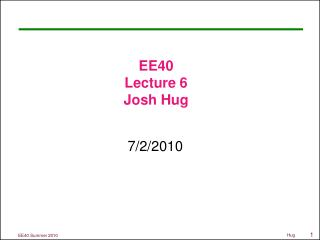Download PresentationEE40 Lecture 6 Josh Hug

# EE40 Lecture 6 Josh Hug - PowerPoint PPT PresentationDownload Presentation## EE40 Lecture 6 Josh Hug

- - - - - - - - - - - - - - - - - - - - - - - - - - - E N D - - - - - - - - - - - - - - - - - - - - - - - - - - -
##### Presentation Transcript

1. EE40Lecture 6Josh Hug 7/2/2010

2. General Info • HW2 due today at 5PM • HW3 out, due TUESDAY at 2PM • There will be an optional pre-midterm HW available Tuesday • No lecture Monday • Labs as usual on Tuesday • No lab Wednesday • Midterm next Fridayin class • 12:10-1:30 [be on time!] • No electronic devices • One 8.5”x11” (or A4) sheet of paper • Handwritten anything you want, both sides

3. Op-Amps – How Good Are They Exactly? • We’ve been studying ideal op-amps • Of course, real Op-Amps aren’t perfect • For example, you can’t drive every device in the universe from a real op-amp • How do we precisely state the quality of a voltage source? • Look at its Thevenin equivalent • Lower Thevenin resistance is better

4. Example: Batteries • Real voltage sources, like batteries, have a limit to how much current they can draw • Called “internal resistance” • This internal resistance often varies with charge status, load attached, temperature, and more • Just like Thevenin resistance e.g., a car battery supplies 12 Volts, and can supply at most 200 amps, what is its internal resistance? RTH + VTH Vout – + – 12V/200A=0.06Ω

5. Measuring the Quality of a Source • If you attach a resistive load, then the output voltage is: • If you want Vout to be 99% of VTH, then: So basically, for loads which are more than 99 times the Thevenin resistance, you get >99% of the Thevenin voltage RTH + VTH Vout – + RL – Lower RTH is better, can handle smaller loads

6. Source Quality Example • Everyone’s favorite resistive power supply again, vo=vi/1000 Vout RL Vin 2/3Ω a 1/1000V – + RL=99*2/3Ω=66Ω 66Ω load gets 99% of VTH b

7. Thevenin Equivalents of Op-Amp circuits • Can find Thevenin equivalent of an op-amp circuit at its output terminals: • Just like finding Thevenin equivalent of a simple resistor based voltage attenuator at its output terminals: RTH vo VTH – + 2/3Ω a 1/1000V – +

8. Thevenin of Inverting Amplifier • Assuming that the op-amp here is IDEAL, what’s the best way to find the Thevenin equivalent circuit? • We’ve already derived that it’s a perfect voltage source! RTH vo VTH – +

9. But if you really want to… Technically you should take limits here but we are lazy…

10. What’s wrong? • Our op-amp model is missing something • That’s why it’s the “ideal” op-amp model • We’ll now introduce the “resistive” op-amp model Resistive Op-Amp Model Ideal

11. Resistive Op-Amp model • Takes in to account the fact that • Some current flows into the input terminals • The op-amp cannot source all device in the universe (output resistance is non-zero)

12. Output Resistance of Inverting Op-Amp • On board (using resistive model of op-amp) • Output Resistance • Tells us how small our load can be before we start losing signal fidelity

13. Input Resistance • Resistance at the input terminals of a device • Tells us how much current will be generated for a fixed input voltage • Useful, for example, to find power needed to power a device (at that input)

14. Input Resistance of Inverting Amplifier • What is the input resistance of an inverting amplifier using ideal op-amp model? • What is the input resistance of an inverting amplifier using resistive op-amp model? (See sec 15.42 in book)

15. Why are these quantities useful? • Input resistance tells us how much current (power) our input signal needs to provide • Output resistance says how small of a load we can drive

16. Why are these quantities useful? • An iPod provides roughly 1V signal output with 20Ω internal resistance • Speakers might be 4Ω resistance • Connect iPod directly to such speakers • Internal resistance dominates

17. Why are these quantities useful? 20Ω 1V – + Rs – + 4Ω iPod Speakers

18. Bad Amplifier (Small Rs) • Very small Rs • iPod must supply 50mW • Output resistance is large (can’t drive speakers) 20Ω 1V – + Rs – + 4Ω iPod Speakers

19. Good Amplifier • Rs=1000, Rf=3000, A=106, Rt=1000 20Ω 0.00133Ω 1V – + Rs – + 4Ω 2.93V iPod Speakers Must provide 1 mW

20. Op-Amp Saturation • Remember those power ports we’ve been ignoring? • They specify the maximum and minimum voltage that our op-amp can deliver • If • Op-Amp output is • If , • Op-Amp output is • If , • Op-Amp output is

21. Op-Amp Saturation Example • , • If A=3: 12V 4V -4V -12V

22. Positive Feedback On the board

23. That’s all forOp-Ampsks • No class Monday • Enjoy weekend (doing op-amp problems)